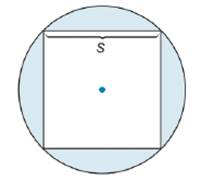Chapter 8.5, Problem 33EElementary Geometry For College St...

7th Edition
Alexander + 2 others
ISBN: 9781337614085

Solutions

Chapter
SectionElementary Geometry For College St...

7th Edition
Alexander + 2 others
ISBN: 9781337614085
Textbook Problem

Determine a formula for the area of the shaded region determined by the circle and its inscribed square.To determine

To find:

A formula for area of shaded region.

Explanation

Formula:

Area of circle:

If r is the radius of circle, then area of circle is given by the formula:

Acircle=πr2.

Area of square:

If the length of side of a square is a, then the area of square is given by the formula:

Asquare=a2.

Pythagoras theorem:

In a right angled triangle, if the length of hypotenuse is c and the length of remaining two sides of triangle is a and b, then by Pythagoras theorem, c2=a2+b2.

Calculation:

The area of shaded region can be determined by subtracting the area of inscribed square from the area of circle.

The length of side of square is given as s.

Thus, Asquare=s2

From the diagram, it is clear that the diameter of circle is equal to the diagonal of square.

Let the length of diagonal be d.

All the angles of a square are equal and they measure 900. Thus, the diagonal of square and a pair of adjacent sides form a right angled triangle.

The length of diagonal of the square can be found by using Pythagoras theorem.

The diagonal is the hypotenuse of right angled triangle.

d2=a2+b2

Here, the lengths of remaining two sides of triangle are equal to the side of square. Thus, a=b=s. Let’s substitute s in place of a and b.

d2=s2+s2

d2=2s2

Taking square root on both sides,

d=2×s

Hence, the length of diameter of circle =2×s

We know that, radius of a circle is half of the length of diameter

Still sussing out bartleby?

Check out a sample textbook solution.

See a sample solution

The Solution to Your Study Problems

Bartleby provides explanations to thousands of textbook problems written by our experts, many with advanced degrees!

Get Started

Show that sin-1(tanh x) = tan1(sinh x).

Single Variable Calculus: Early Transcendentals, Volume I

Evaluate the indefinite integral. sinh2xcoshxdx

Single Variable Calculus: Early Transcendentals

limx0cscx= a) 0 b) c) d) does not exist

Study Guide for Stewart's Single Variable Calculus: Early Transcendentals, 8th

Which curve is simple but not closed?

Study Guide for Stewart's Multivariable Calculus, 8th